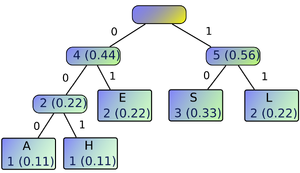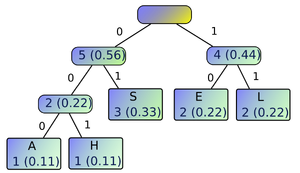269 PagesWikipedia has an article related to: Huffman coding

In computer science and information theory, Huffman coding is an entropy encoding algorithm used for lossless data compression. The term refers to the use of a variable-length code table for encoding a source symbol (such as a character in a file) where the variable-length code table has been derived in a particular way based on the estimated probability of occurrence for each possible value of the source symbol. It was developed by David A. Huffman while he was a Ph.D. student at Massachusetts Institute of Technology, and published in the 1952 paper "A Method for the Construction of Minimum-Redundancy Codes".

Huffman coding uses a specific method for choosing the representation for each symbol, resulting in a prefix code (that is, the bit string representing some particular symbol is never a prefix of the bit string representing any other symbol) that expresses the most common characters using shorter strings of bits than are used for less common source symbols. Huffman was able to design the most efficient compression method of this type: no other mapping of individual source symbols to unique strings of bits will produce a smaller average output size when the actual symbol frequencies agree with those used to create the code. A method was later found to do this in linear time if input probabilities (also known as weights) are sorted.

For a set of symbols with a uniform probability distribution and a number of members which is a power of two, Huffman coding is equivalent to simple binary block encoding, e.g., ASCII coding. Huffman coding is such a widespread method for creating prefix codes that the term "Huffman code" is widely used as a synonym for "prefix code" even when such a code is not produced by Huffman's algorithm.

Although Huffman coding is optimal for a symbol-by-symbol coding (i.e. a stream of unrelated symbols) with a known input probability distribution, its optimality can sometimes accidentally be over-stated. For example, arithmetic coding and LZW coding often have better compression capability. Both these methods can combine an arbitrary number of symbols for more efficient coding, and generally adapt to the actual input statistics, the latter of which is useful when input probabilities are not precisely known or vary significantly within the stream. In general, improvements arise from input symbols being related (cat is more common than cta).

## Algorithm

The algorithm basically encodes a string of symbols (example: strings of characters or bytes in a file) into a prefix code (an optimal Huffman code) of codewords (symbols used in the encoding of the symbols) (made up of symbols from an alphabet) with the minimum expected codeword length (and consequently encoded string length) using a tree (a tree is an acyclic connected graph of points) constructed from the weights of the symbols, to encode and to decode.

### Creating a Huffman tree

The first step to the algorithm is constructing a Huffman tree. Symbols that appear more often will come "higher", that is, closer to the root node, in the tree, and will have shorter codewords; those that appear less often will come "lower" in the tree, and will have longer codewords.

Steps in constructing a binary Huffman tree:

1. Determine the probability (or frequency) of each symbol that occurs in the source code, the code to be encoded. One may start by counting or tallying them and placing the results in a table.
2. Sort the symbols by their probability (or frequency). (Sorting will finish the algorithm in linear time.)
3. If there are only two symbols (or root nodes of previously constructed trees, if any), construct a tree with a root node (you can assign the probability 1 if you like) with the symbols as nodes, the value of one path to one symbol being 0 and the other being 1.
4. Take the two symbols (or root nodes of previously constructed trees, if any) with the smallest frequencies or probabilities (weights) and construct a tree with the root node having a weight equal to the sum of the the weights of the symbols and the nodes as the symbols, the value of one path to one symbol being 0 and the other being 1.
5. Repeat step 3 until only one tree remains.

For example, in constructing the Huffman tree for the string "SEASHELLS":An optimal Huffman tree constructed from the string "SEASHELLS". The corresponding Huffman code encoded from this string with this tree is 100100010001011111.Another optimal Huffman tree constructed from the string "SEASHELLS". The corresponding Huffman code encoded from this string with this tree is 011000001001101111, which has the same length as the previous tree, 17 characters.
• Frequency of symbols (each character is a symbol):
Symbol (sorted by appearance in string) Frequency
sorta_S 3
sortb_E 2
sortc_A 1
sortd_H 1
sorte_L 2
• Constructing the tree, starting from A(1) and H(1). We will consider the left node as 0 and the right node as 1.
• [A(1) , H(1)](2) - 2 being the sum of the weights of A and H.
• Taking E(2) and the previous tree. Note that we can also take L(2), but any tree constructed with this algorithm is optimal anyway (as optimal as any other tree with any chosen combination).
• [A(1) , H(1)](2) , E(2)](4)
• Taking S(3) and L(2), since the previous tree already has a weight of 4, and these nodes have smaller weights than the tree.
• [S(3) , L(2)](5)
• Finally, we have two nodes left.
• [[A(1) , H(1)](2) , E(2)](4) , [S(3) , L(2)](5)]

### Encoding and decoding

Using a Huffman tree (optimal or not), one may determine the codeword for a symbol (encoding) by looking for the symbol in the tree then following through the tree from the root node to the node of the symbol, taking note of the path values taken. The resulting string of path values is the codeword.

For example, to determine the code for "S" using the example tree in the previous section, starting from the root node, we go right (1), then left (0); the code is "10".

We can just concatenate these codes since the tree is a prefix code. For example, the code for "SHE" is "10" + "001" + "01" = "1000101".

Likewise, one may determine the symbol for a string of codewords (decoding) by following through the tree, following the path determined by the character in the string of codewords until a symbol is encountered; and the act of following goes back to the root node in case more codewords are to be decoded.

For example, to decode the string "001011111", we start with the root node, then left (0), left (0), then right (1). We encounter a symbol ("H"), so we go back to the root node. The string decodes to "HELL".

### Miscellaneous

Miscellaneous problems in Huffman coding include encoding the Huffman tree itself to be used to decode or a table or dictionary of symbols and the corresponding codeword (we don't need to encode the weights of the nodes in the tree). Since the codewords are of variable length, the length of each codeword must also be stored if it is to be stored in a byte (8-bit) form.

As an example implementation, one can encode the tree with just the symbols {internal node, leaf node [codeword length, n-bit] [codeword], end of tree} in prefix form. We also take note of the maximum codeword length. The Huffman tree need not be optimal. An example table would be:

Symbol Code
leaf node 0
internal node 10
end of tree 11

Example coding of sample implementation:

 Maximum codeword length, 8-bit: 3 00000011 Start of tree: internal node 10 Symbol (1 - 4-bit) 0 0001 Internal node 10 Symbol (3) 0 0011 Symbol (2) 0 0010 End of tree 11

## Variations

• n-ary Huffman coding - uses numbers from 0 to n. In the algorithm, n symbols are selected instead of just two in binary Huffman coding. In the special case when the number of symbols r is less than or equal to n, only the first r numbers are used to construct the tree.
• Adaptive Huffman coding - computes the probabilities dynamically as the string is encoded.
• Canonical Huffman coding - If weights corresponding to the alphabetically ordered inputs are in numerical order, the Huffman code has the same lengths as the optimal alphabetic code, which can be found from calculating these lengths, rendering Hu-Tucker coding unnecessary. The code resulting from numerically (re-)ordered input is sometimes called the canonical Huffman code and is often the code used in practice, due to ease of encoding/decoding. The technique for finding this code is sometimes called Huffman-Shannon-Fano coding, since it is optimal like Huffman coding, but alphabetic in weight probability, like Shannon-Fano coding. The Huffman-Shannon-Fano code corresponding to the example is {000,001,01,10,11}, which, having the same codeword lengths as the original solution, is also optimal.

## See also

 This page uses CC-BY-SA content from Wikipedia (authors).Community content is available under CC-BY-SA unless otherwise noted.